## 4.6 Exercises### 4.6.1 Good vs. bad examples

1. Find and collect a good vs. a bad example of a plot (e.g., in brochures, newspapers, media reports, scientific articles, etc.).
• What makes the good one good?
• How could the bad one be improved?

Hint: There are many great sources for inspiration. For instance, check out r/dataisbeautiful at reddit.com.

1. Bonus:13 Create a misleading and a transparent visualization for the same data.

### 4.6.2 Plot types

Evaluate and compare the following commands in R:

• plot(Nile)
• plot(cars)
• plot(iris)
• plot(Titanic)

Can you explain the types of the resulting plots in terms of the data provided?

#### Solution

The type of plot automatically chosen by R depends on the data provided to the plot() function (see Figure 4.10):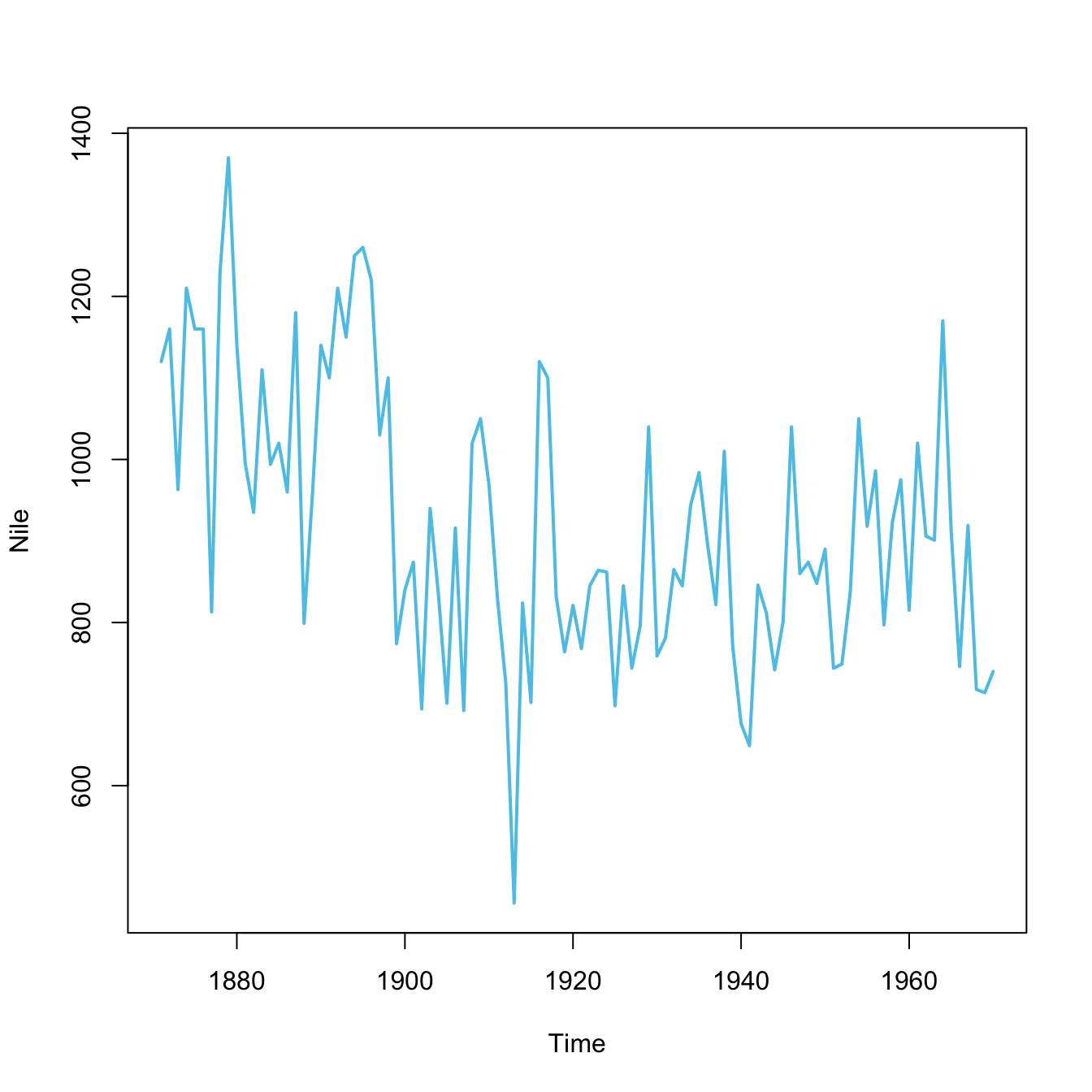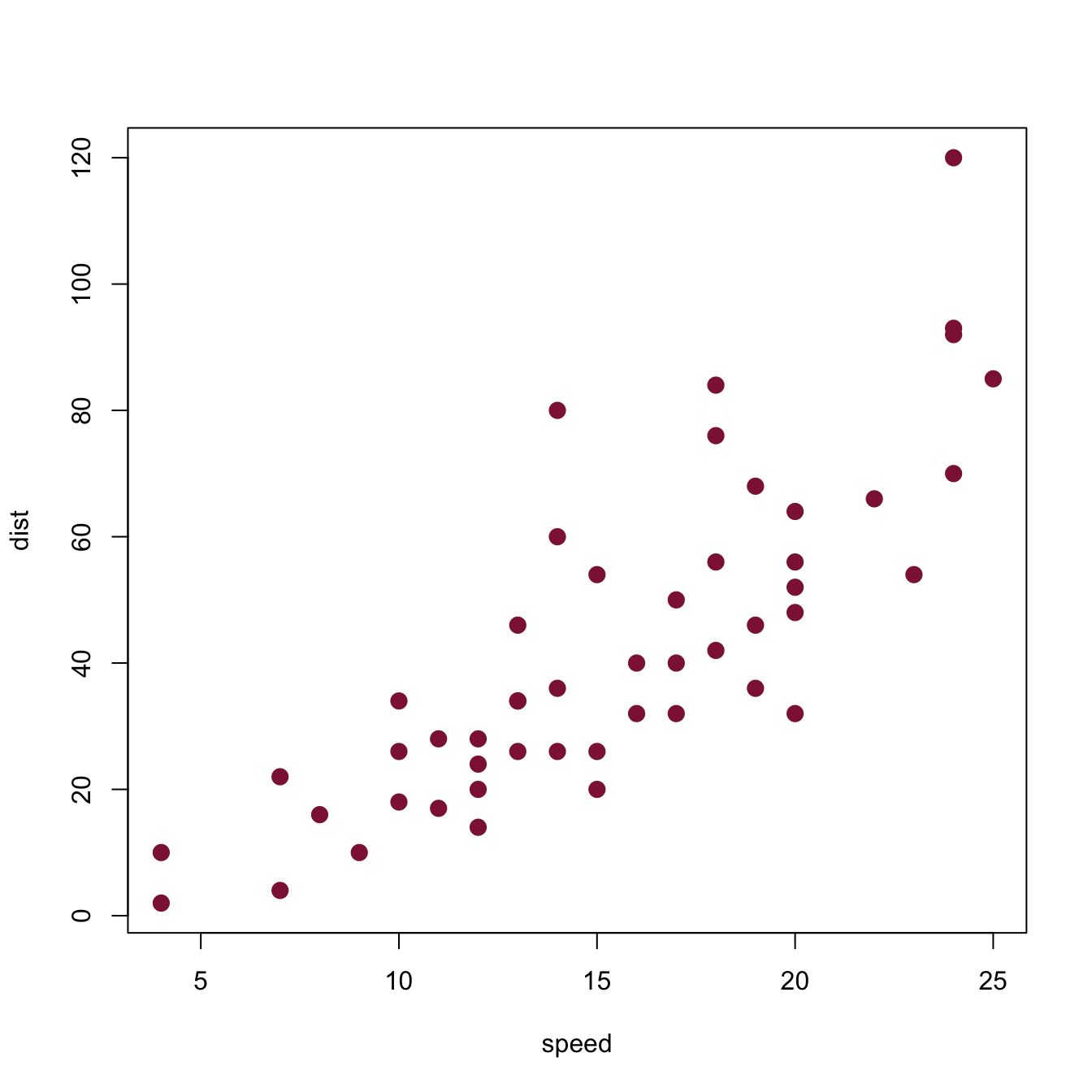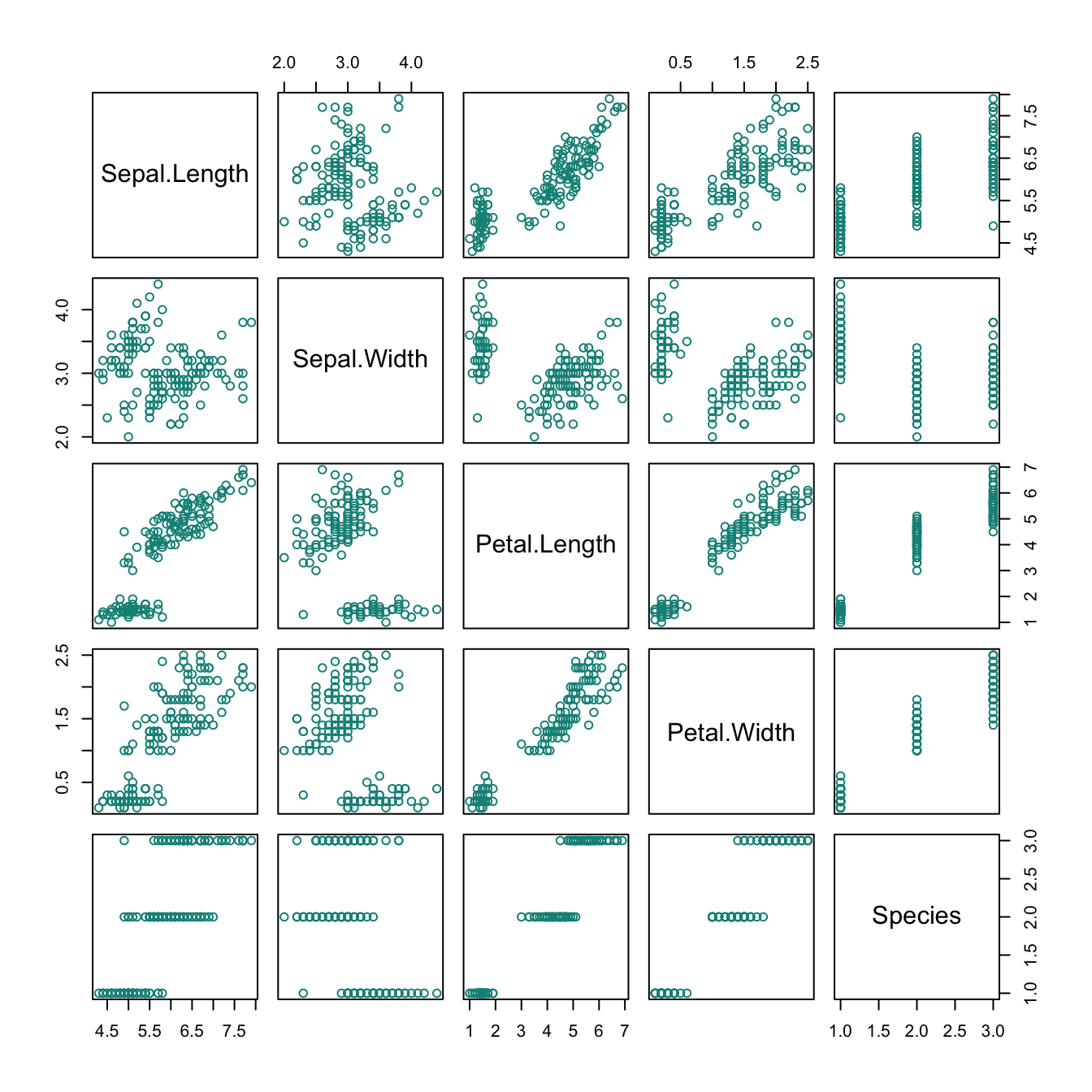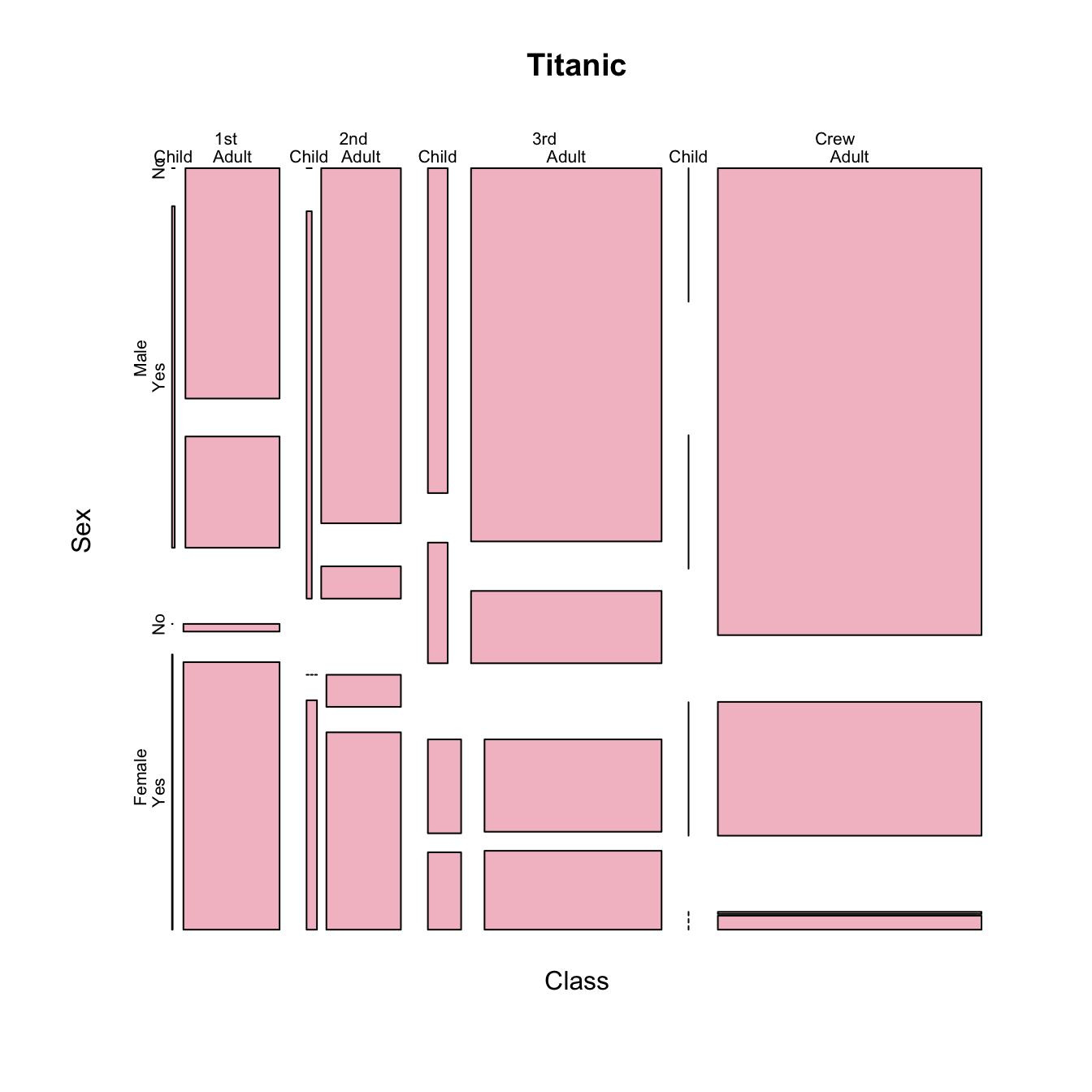Figure 4.10: Plots created by calling plot(x) with different types of objects x.

• plot(Nile) plots a time series as a line plot.
• plot(cars) plots a data frame of 50 observations and 2 variables as a scatterplot.
• plot(iris) plots a data frame with 150 cases and 5 variables (4 numeric, 1 character) as 5x5 scatterplots.
• plot(Titanic) plots the counts of 4 categorical variables as a (complex) mosaic plot.

### 4.6.3 Plotting the Nile

Plot the Nile data and justify your choice of plot.

#### Solution

Note that Nile data is a time series.

Figure 4.11 shows some options.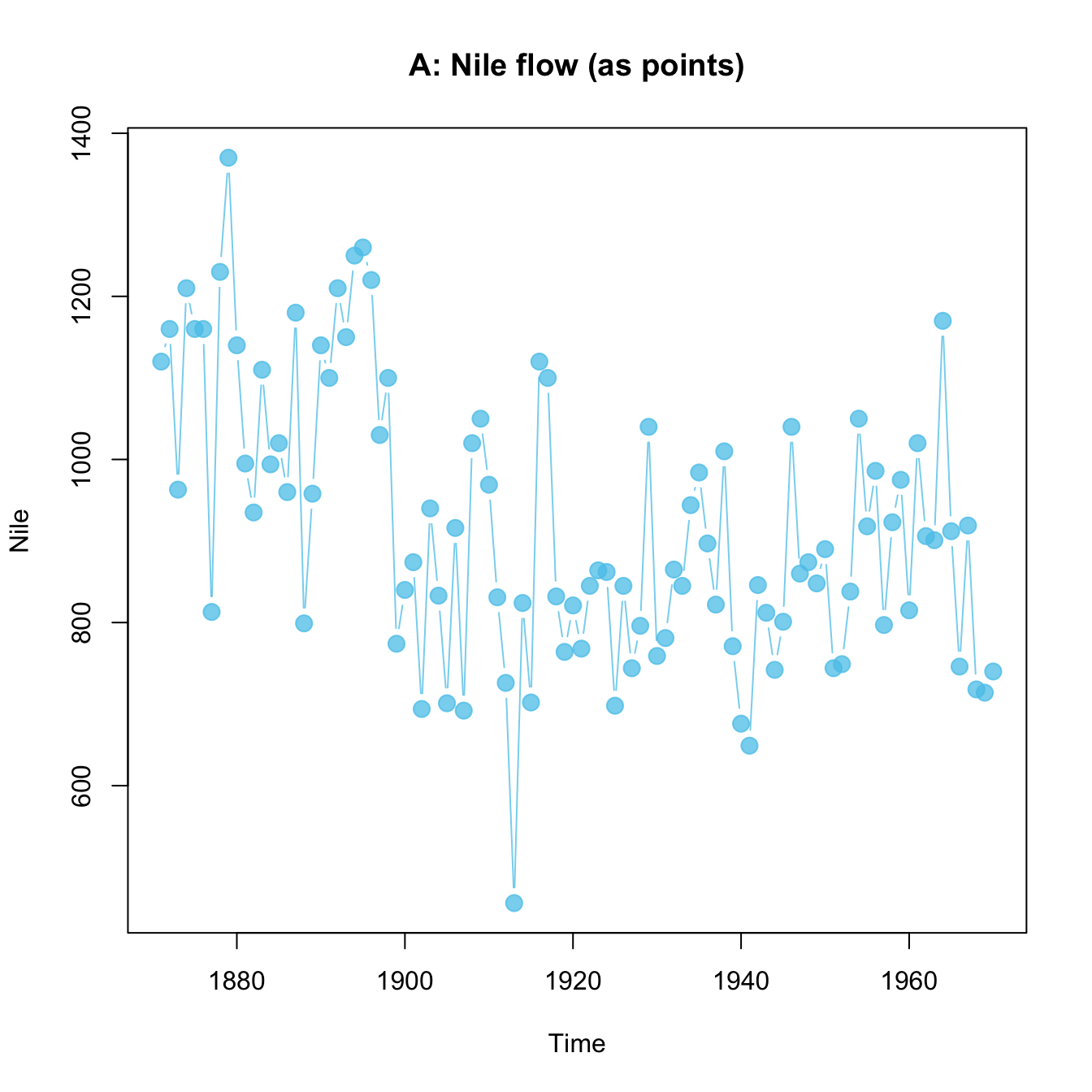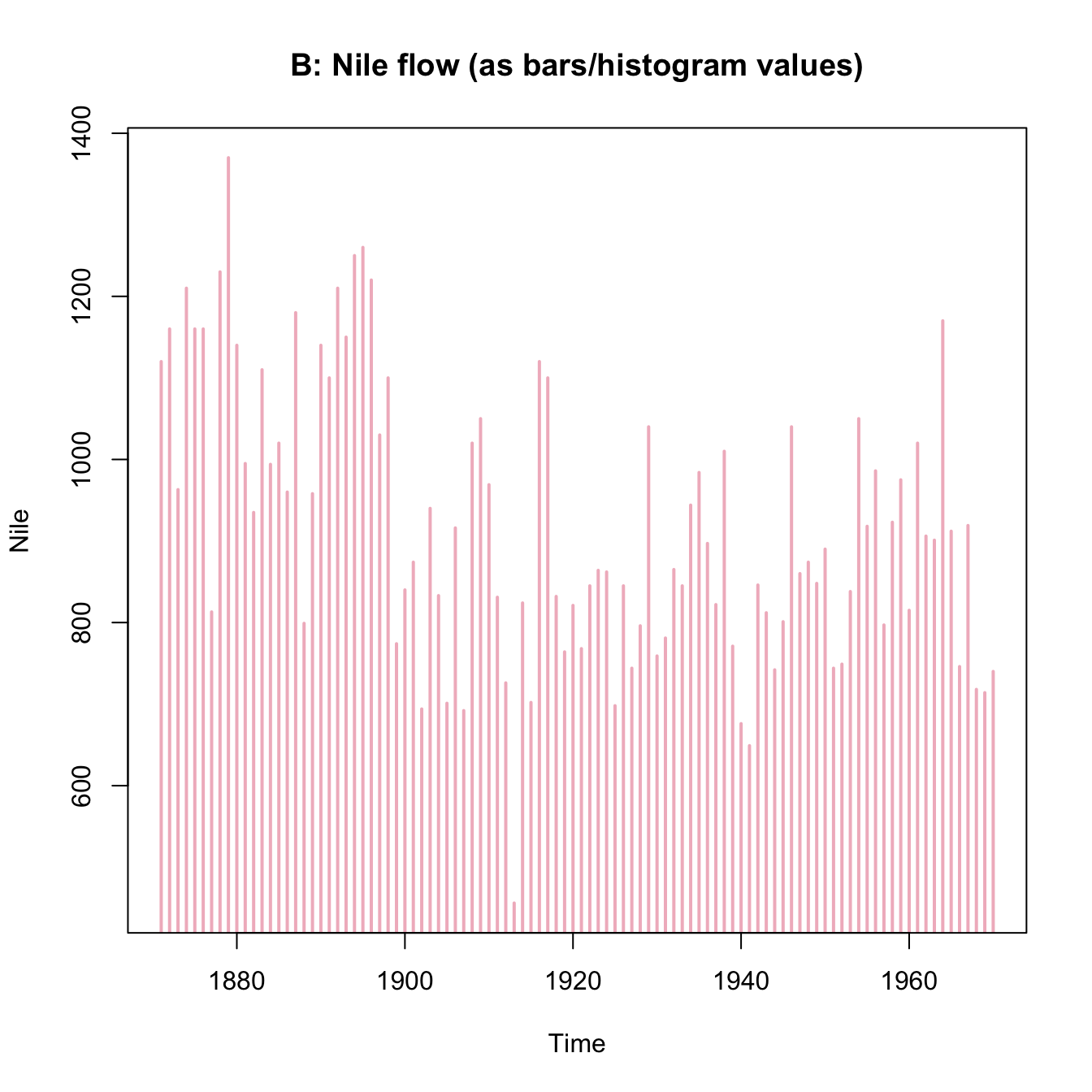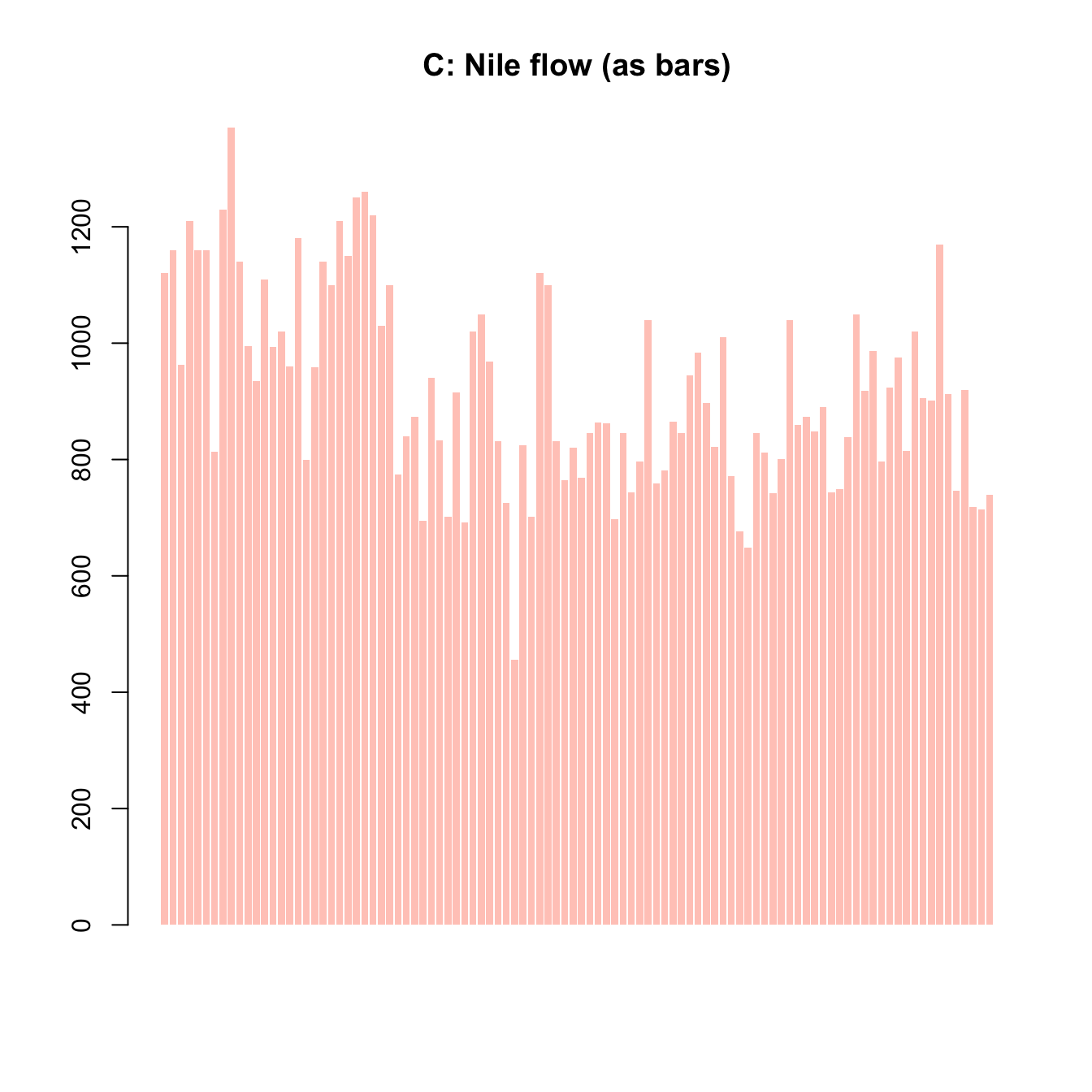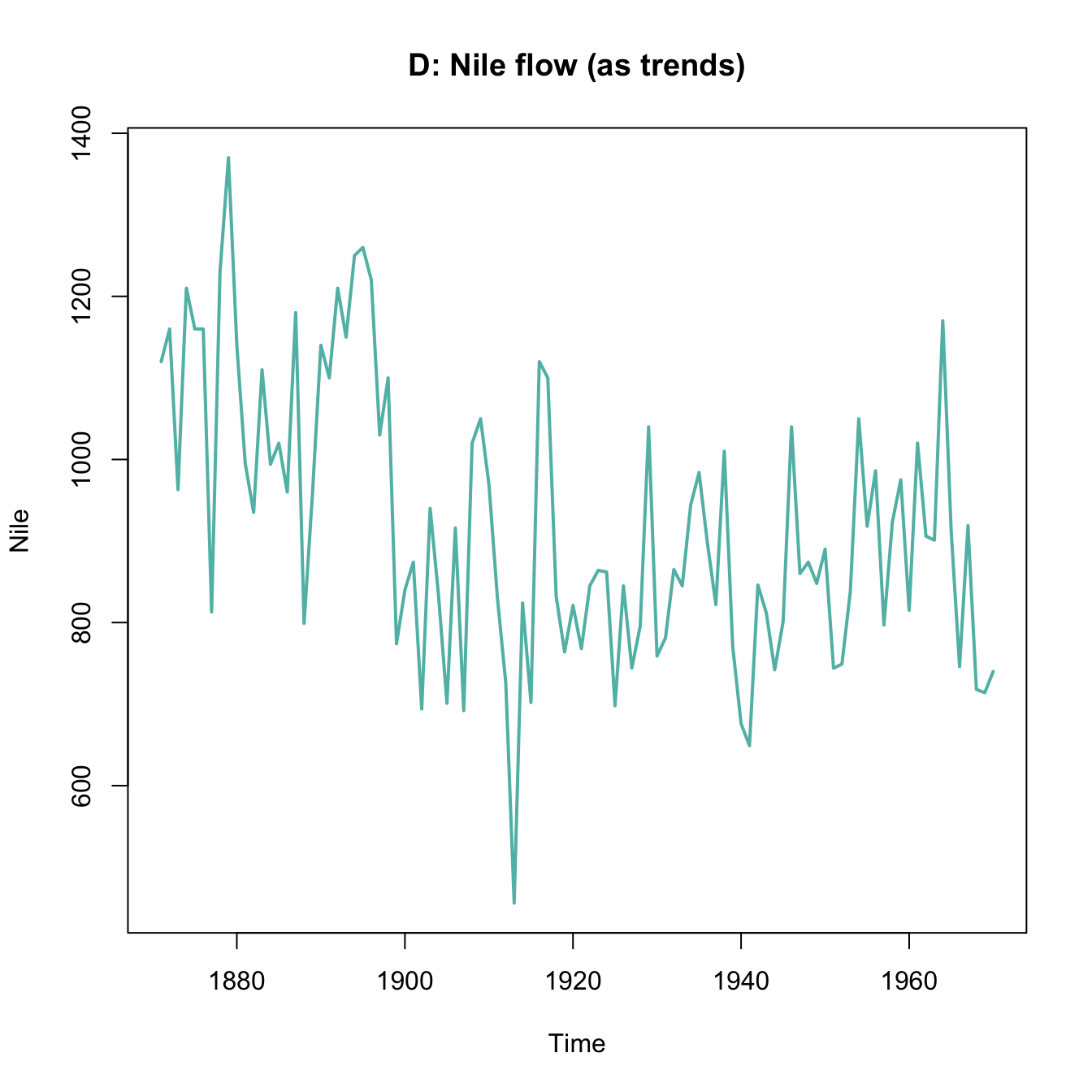Figure 4.11: Various ways of plotting the Nile data emphasizes different aspects.

### 4.6.4 Plotting a histogram

Using the mpg data from the ggplot2 package, create a histogram that shows the distribution of values of the cty variable (showing a car’s fuel consumption, in miles per gallon (MPG), in the city).

Getting the data:

mpg <- ggplot2::mpg

Before starting to plot anything, we should always first inspect and try to understand our data:

# Print data table:
mpg  # a tibble with 234 cars, 11 variables

# We are interested in the vector
mpg$cty # Note: ?mpg # describes the data #### Solution Here is how your histogram could look like: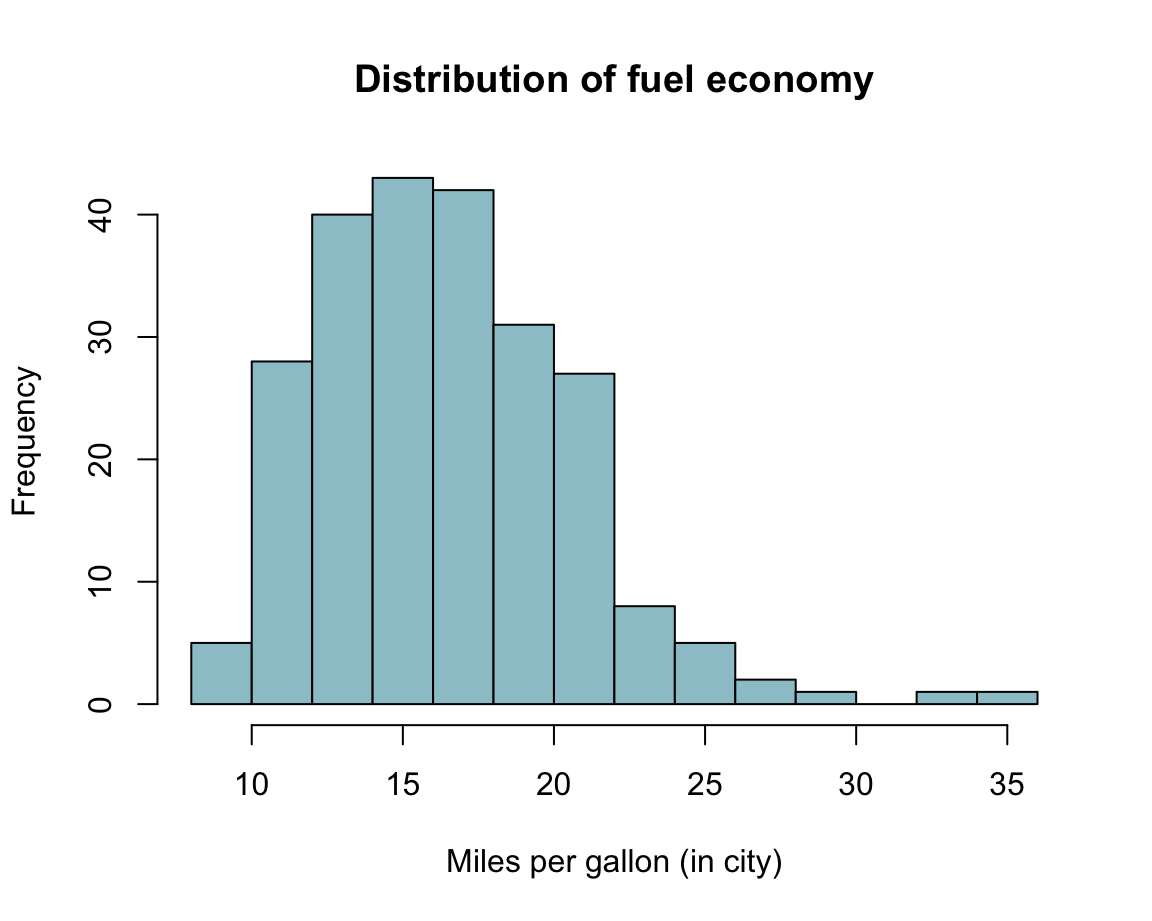### 4.6.5 Plotting a scatterplot Using variables from data: A typical scatterplot (using the mpg data from ggplot2): mpg <- ggplot2::mpg Create a scatterplot of this data that shows the relation between each car’s • On x-axis: engine displacement (i.e., variable displ of the mpg data), and • On y-axis: fuel consumption on highways (i.e., variable hwy of the mpg data). Can you avoid overplotting? #### Solution Here is how a solution could look like: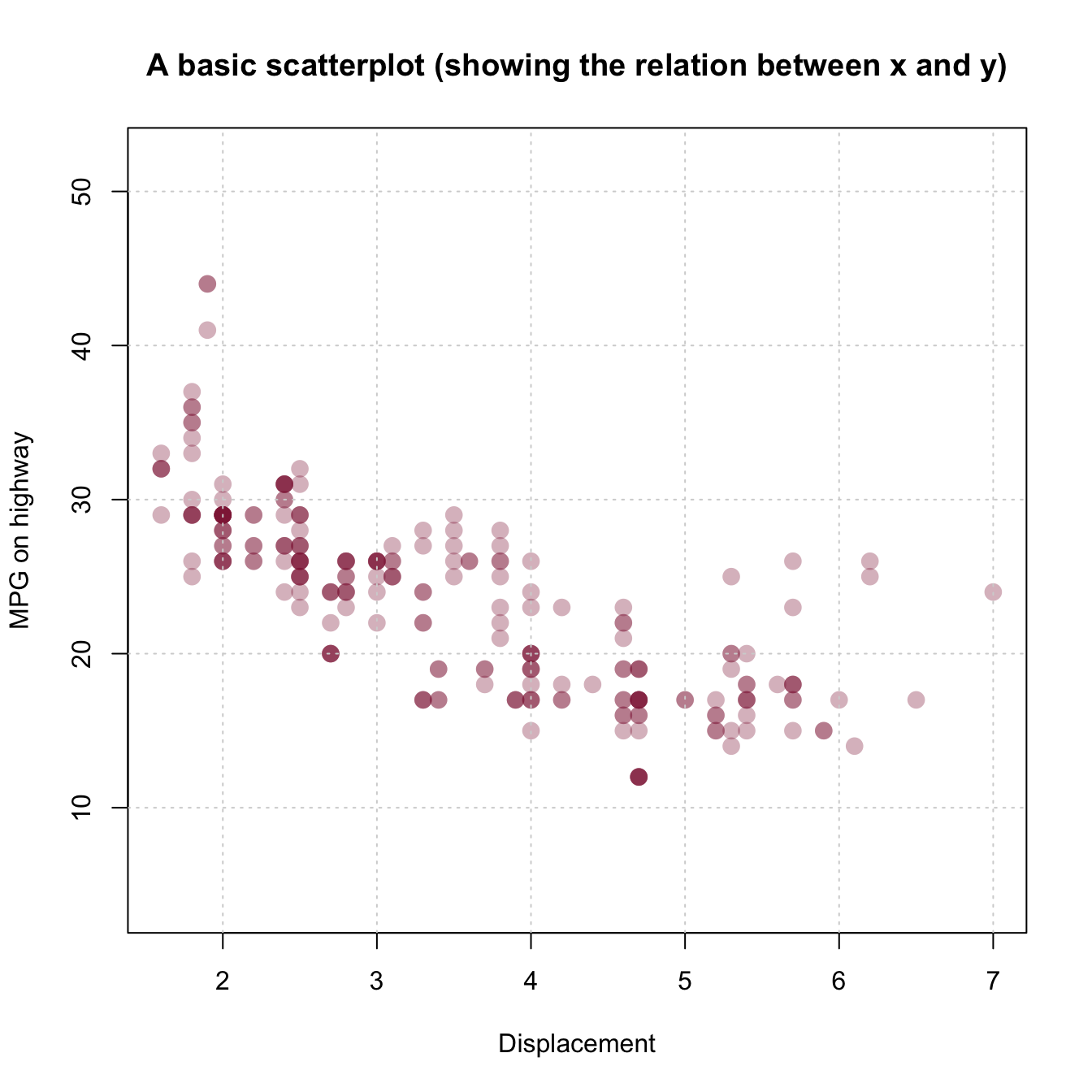### 4.6.6 Plotting bar plots (of election results) In the Practice task of Section 4.3.3, we plotted the share of votes for the two most popular parties of the German Federal elections of 2013 and 2017. 1. Include the data from Bundestag election 2021 to plot the corresponding results for three elections (from 2013 to 2021): 1. with stacked bars (i.e., one bar per year); 1. with bars beside each other (i.e., three bars per year). Here is the data (as a data frame/tidy tibble): library(tidyverse) ## (a) Create a tibble of data: de_new <- data.frame( party = c("CDU/CSU", "SPD", "Others"), share_2013 = c((.341 + .074), .257, (1 - (.341 + .074) - .257)), share_2017 = c((.268 + .062), .205, (1 - (.268 + .062) - .205)), share_2021 = c((.189 + .052), .257, (1 - (.189 + .052) - .257)) ) de_new$party <- factor(de_new$party, levels = c("CDU/CSU", "SPD", "Others")) # optional # de_new ## Check that columns add to 100: # sum(de_new$share_2013)  # => 1 (qed)
# sum(de_new$share_2017) # => 1 (qed) # sum(de_new$share_2021)  # => 1 (qed)

## (b) Converting de_new into a tidy tibble:
tb <- de_new %>%
gather(share_2013:share_2021, key = "election", value = "share") %>%
separate(col = "election", into = c("dummy", "year")) %>%
select(year, party, share)

# Choose colors:
my_col <- c("black", "firebrick", "gold")   # three specific colors
# my_col <- sample(x = colors(), size = 3)  # non-partisan alternative

# Show table:
knitr::kable(tb, caption = "Election data (2013--2021).")
Table 4.4: Election data (2013–2021).
year party share
2013 CDU/CSU 0.415
2013 SPD 0.257
2013 Others 0.328
2017 CDU/CSU 0.330
2017 SPD 0.205
2017 Others 0.465
2021 CDU/CSU 0.241
2021 SPD 0.257
2021 Others 0.502

#### Solution

Here is how a solution could look like:

1. with stacked bars (i.e., one bar per year):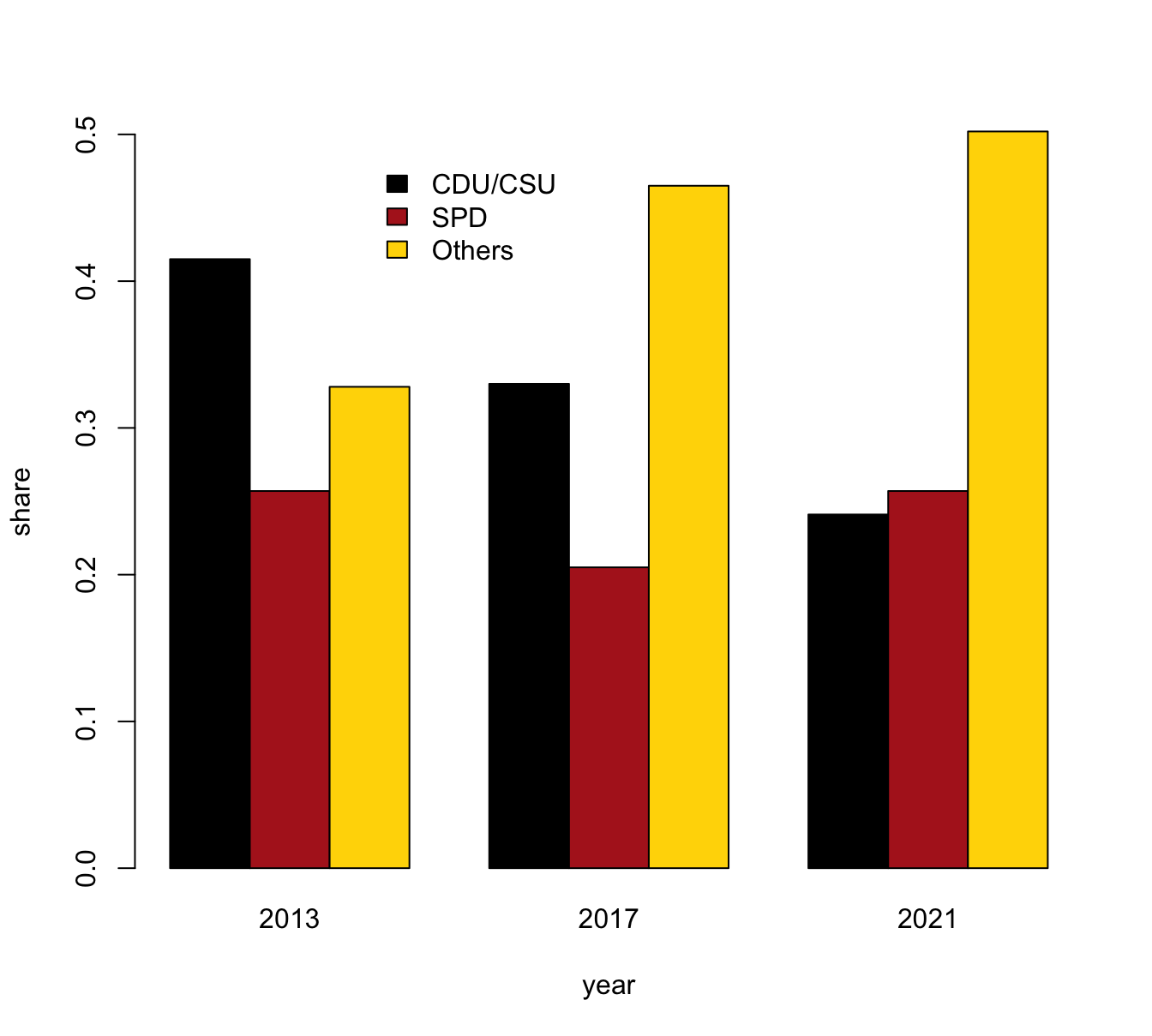1. with bars beside each other: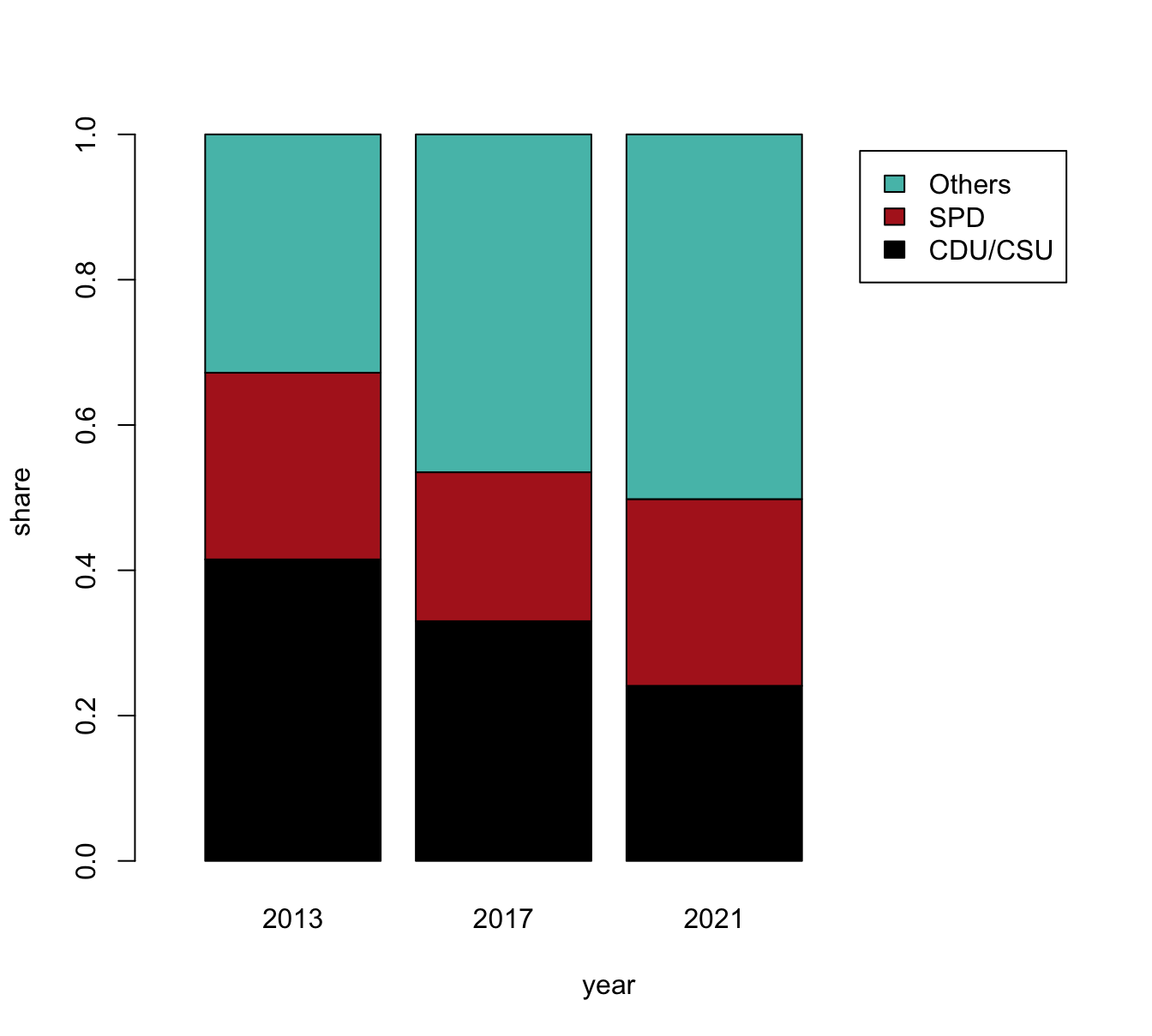Note that the vector my_col was set to three specific colors to facilitate the interpretation of this plot. Interestingly, changing ranks of the third and fourth most popular parties made this choice more difficult for this visualization. Anyone objecting to this choice is welcome to select different colors, or trying out random colors (e.g., by setting my_col to sample(x = colors(), size = 3)).

1. Bonus: Can you reproduce the stacked bar plot showing the Percentage of 2nd votes for all parties?

### 4.6.7 Plotting air quality data

Using the airquality data (included in datasets):

aq <- tibble::as_tibble(datasets::airquality)
1. Create a boxplot and two raw data plots:

Plot the values of Ozone as a function of Month in three ways:

• (a) as a boxplot
• (b) as a raw data plot (with jittered and transparent points)
• (c) as a combination of (a) and (b)
1. Combining scatterplots:

Create three scatterplots of the levels of Ozone by

• (a) Solar.R
• (b) Temp
• (c) Wind

Add a linear regression line for each subplot. Try combining all three plots in one figure.

#### Solution

The following plots show possible solutions:

1. Create a boxplot and two raw data plots:

Plot the values of Ozone as a function of Month in three ways:

• (a) A boxplot: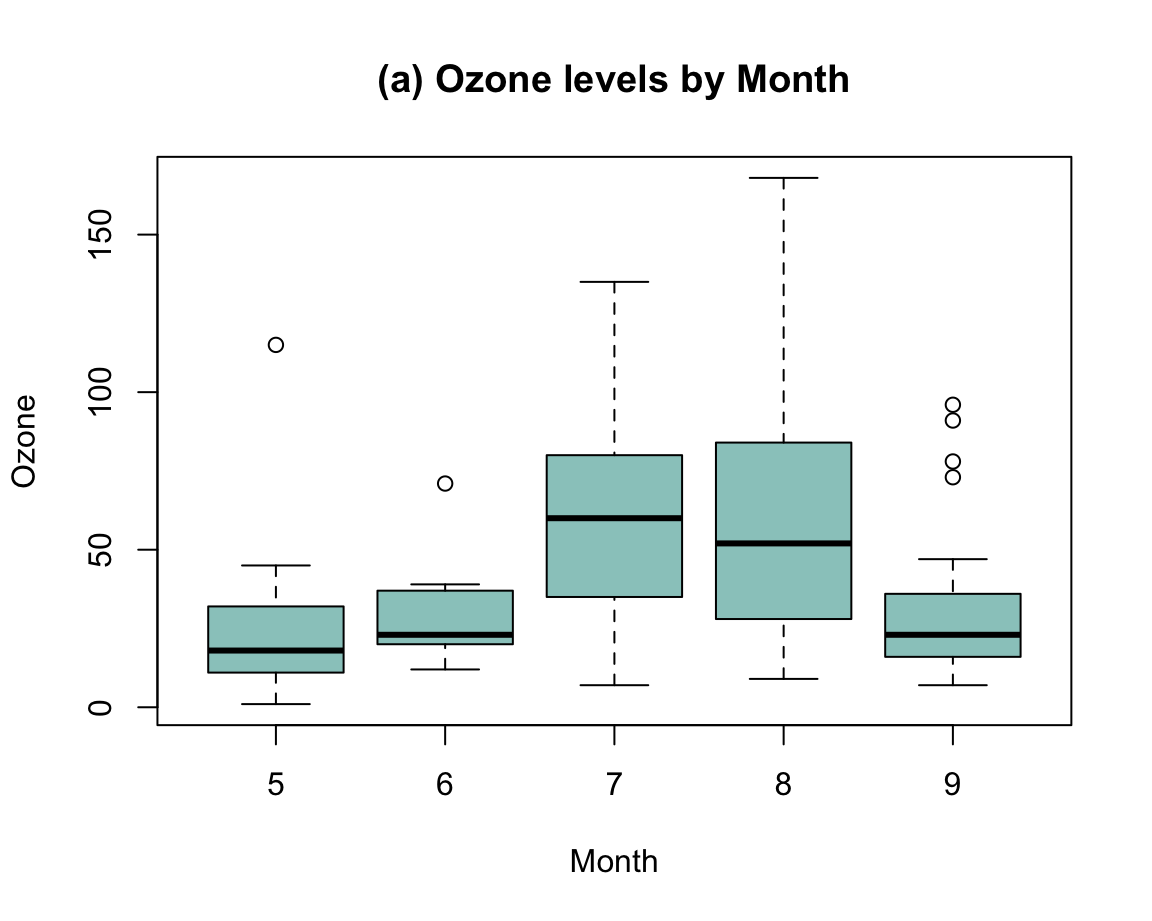• (b) A raw data plot: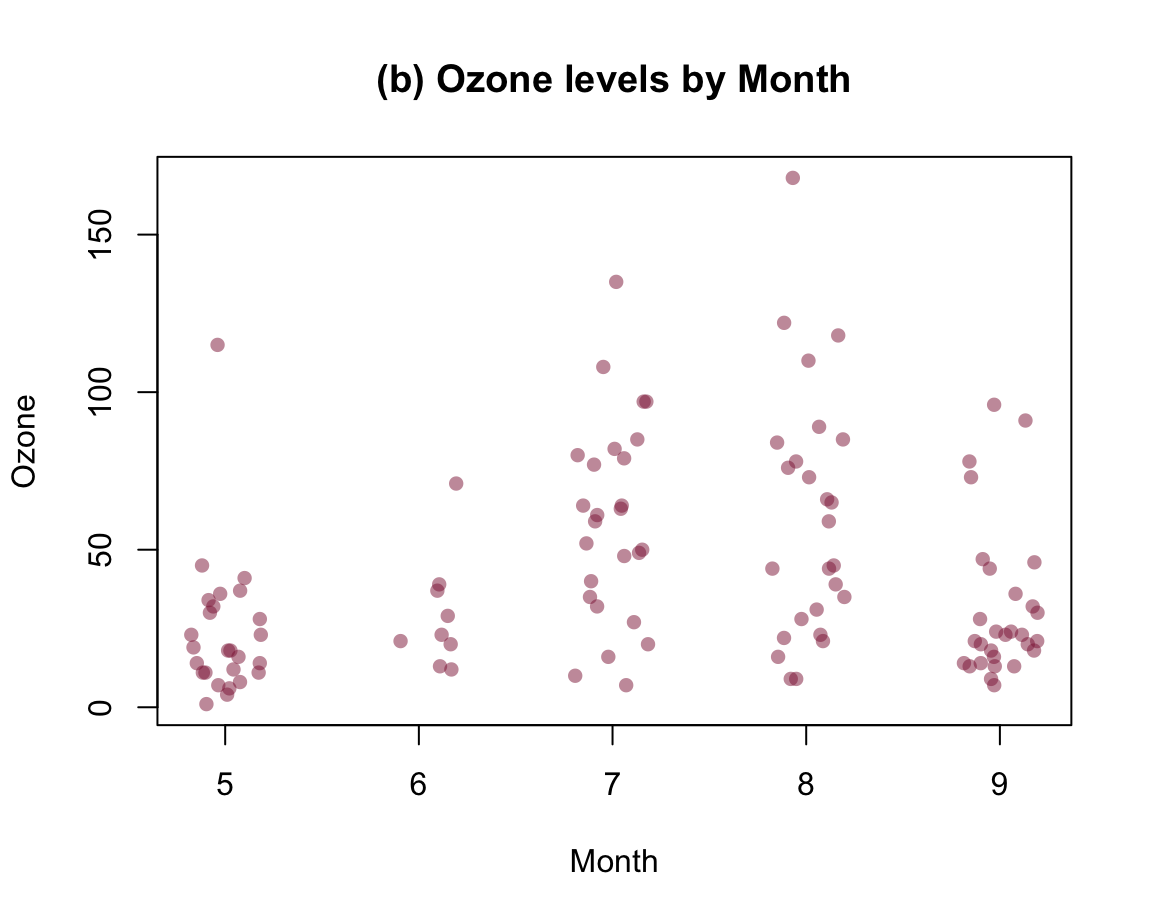• (c) Combination: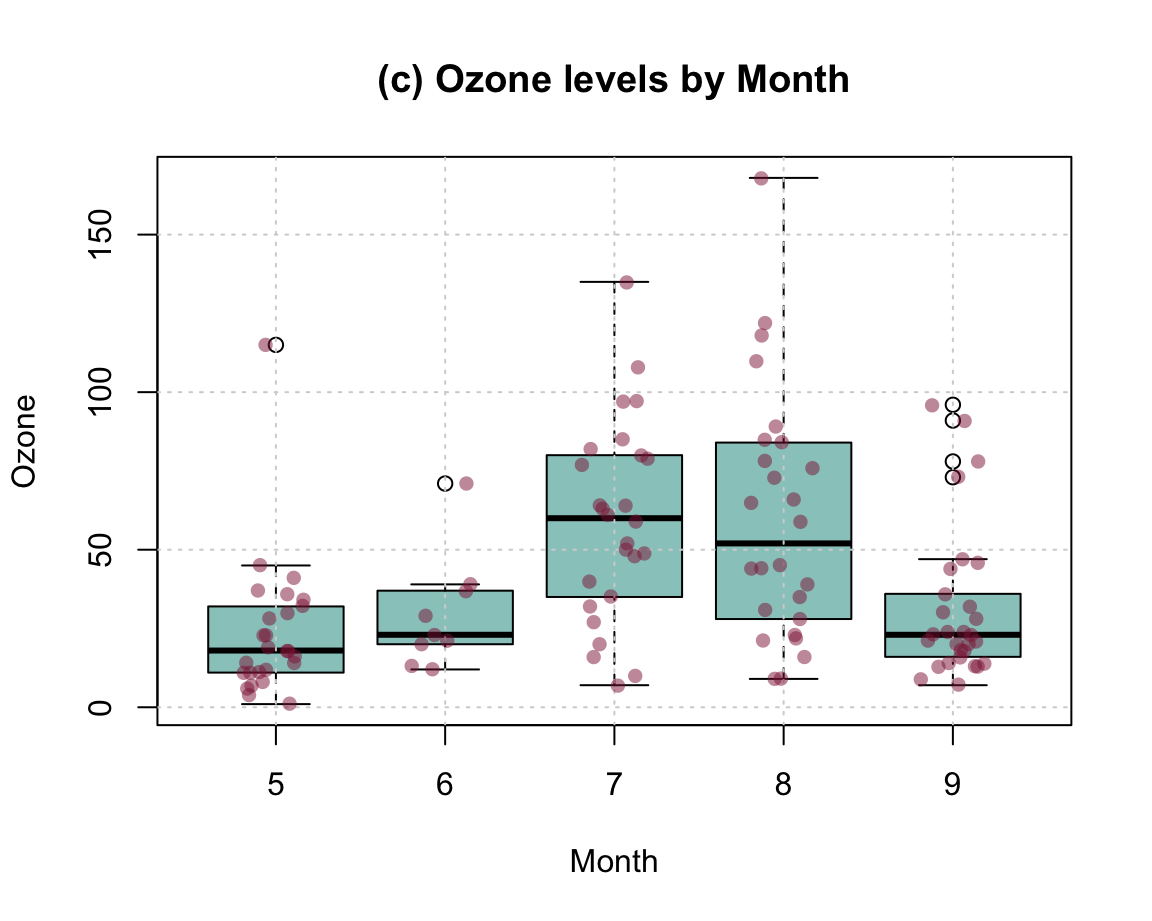1. Combining scatterplots:

Create three scatterplots of the levels of Ozone by

• (a) Solar.R
• (b) Temp
• (c) Wind

Add a linear regression line for each subplot. Try combining all three plots in one figure.

#### Solution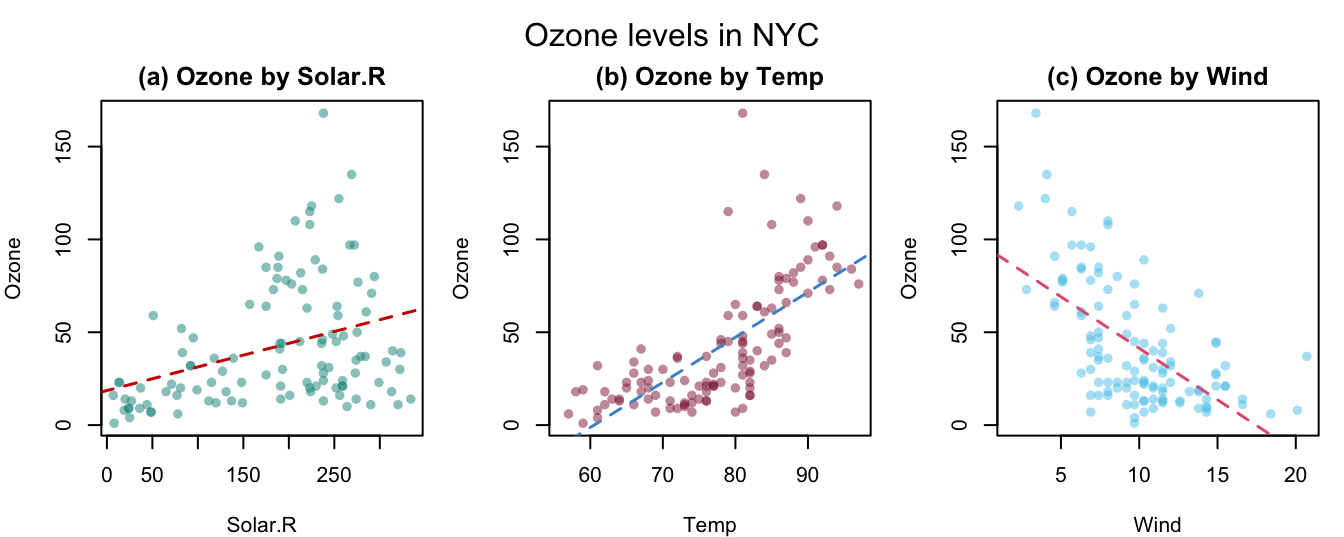### Bonus exercises

The following exercises (marked as Bonus) are optional (i.e., not required for this course).

### 4.6.8 Bonus: Plotting curves (for getting even with percentage changes)

Percentage changes have the peculiar property that gains and losses of the same absolute magnitude differ in their nominal amounts. For instance, when an investment loses $$\frac{1}{4} = 25\%$$ of its original value, it would have to gain $$\frac{1}{3} \approx 33\%$$ to recover its original value.

Use base R to draw a curve that shows the compensatory percentage gain (on the y-axis) for changes from $$-100\%$$ to $$+200\%$$ (on the x-axis).

#### Solution

We first derive an equation that expresses $$y$$ in terms of $$x$$:

• initial value: $$V_0$$
• change by $$x\%$$: $$V_1 = V_0 \cdot (1 + x/100)$$
• change by $$y\%$$: $$V_2 = V_1 \cdot (1 + y/100)$$
• we want that $$V_2 = V_0$$: $$V_0 = V_1 \cdot (1 + y/100) = V_0 \cdot (1 + x/100) \cdot (1 + y/100)$$
• solving for $$y$$ yields: $$y = \frac{100^2}{100+x} - 100$$

The following code implements this equation as an R function and checks it for a vector of values v:

# Function:
y_comp <- function(x){100^2/(100+x) - 100}

# Check:
v <- c(-100, -75, -50, -100/3, -20, -10, 0, 10, 20, 100/3, 50, 75, 100, 200)
y_comp(x = v)
#>          Inf 300.000000 100.000000  50.000000  25.000000  11.111111   0.000000  -9.090909 -16.666667 -25.000000 -33.333333
#>  -42.857143 -50.000000 -66.666667

A corresponding plot could look as follows: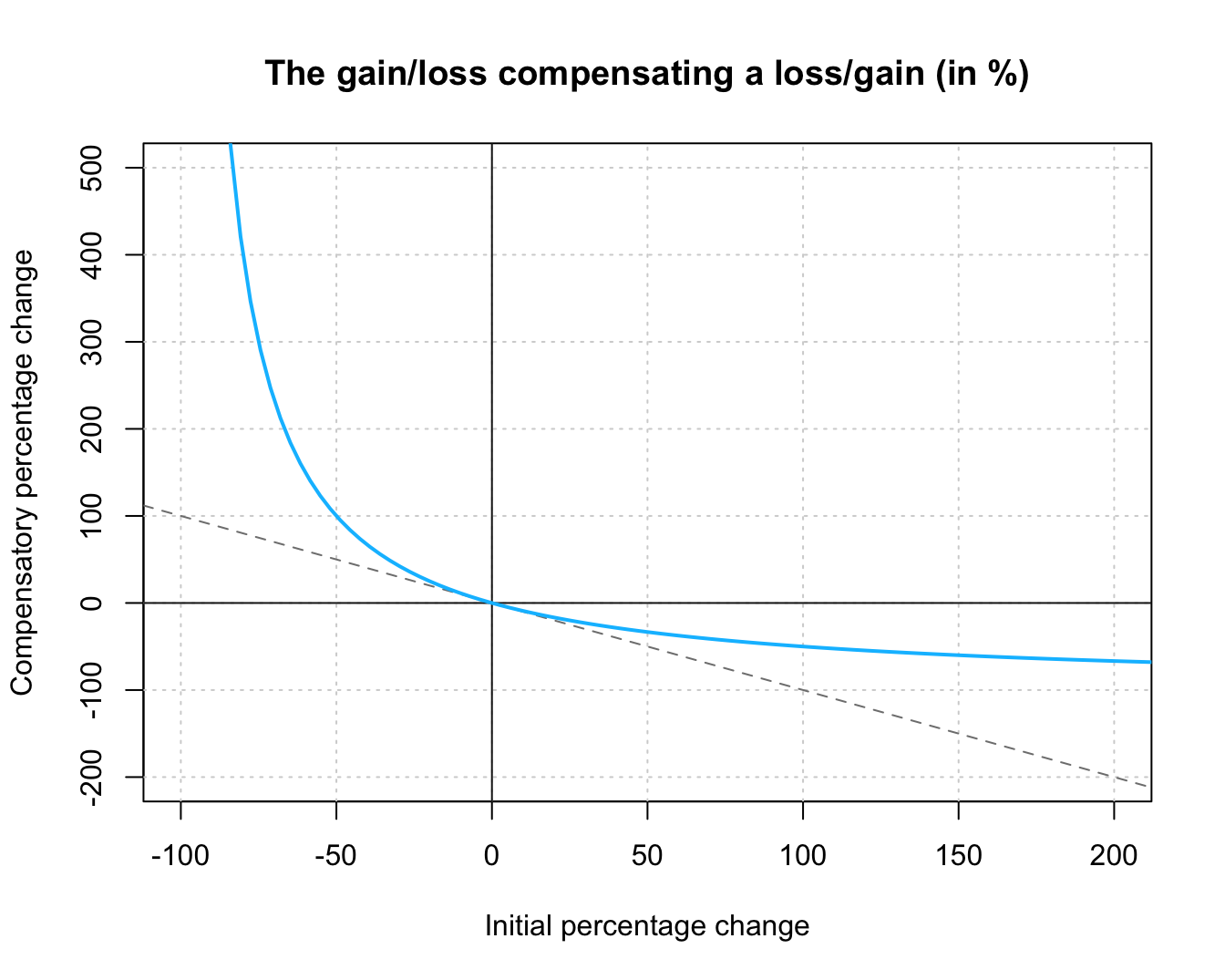Figure 4.12: The percentage gain/loss required for recovering a loss/gain of x%.

Figure 4.12 shows the non-linear relationship between an initial gain/loss (on the $$x$$-axis) and the compensatory loss/gain (on the $$y$$-axis) when both changes are expressed as percentages of the current amount. As the dashed line markes the line where losses and gains were equal, we see that gains by $$x\%$$ are compensated by nominally smaller losses ($$x > |y|$$) and losses by $$x\%$$ are compensated by nominally larger gains ($$|x| < y$$). This implies the counterintuitive fact that first gaining and then losing $$x\%$$ — or vice versa — results in an overall loss.

### 4.6.9 Bonus: Anscombe’s quartet

Re-create the Anscombe plots (shown in Figure 4.1) using the data from datasets::anscombe and base R functions.

#### Solution

Figure 4.13 shows a possible solution: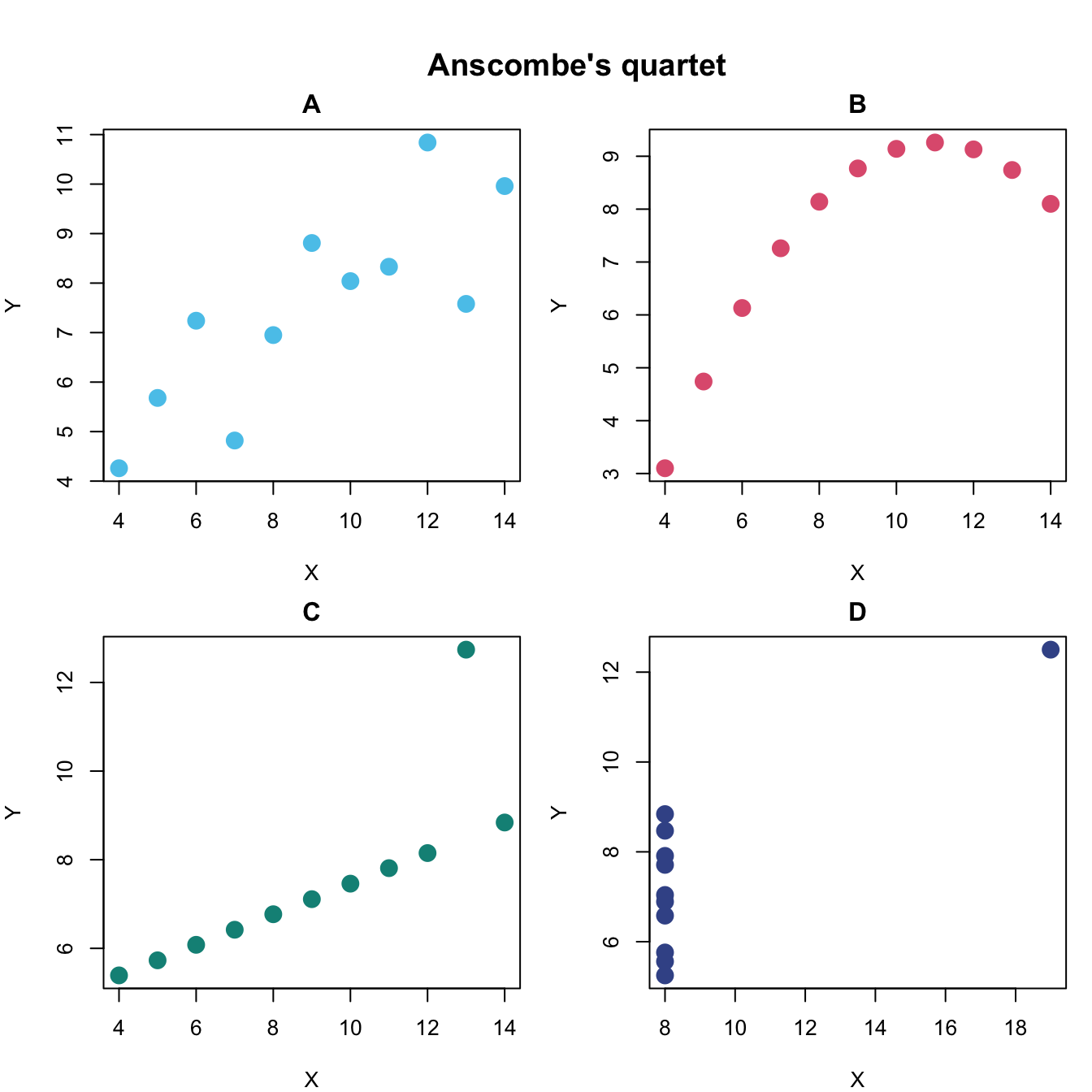Figure 4.13: Scatterplots of Anscombe’s quartet.

Hint: To create Figure 4.13 with base R functions, we need to set the mfrow argument of par() to arrange four plots in two rows and two columns. The four subsets of datasets::anscombe can then be plotted by four calls to plot(x = datasets::anscombe$x1, y = datasets::anscombe$y1), etc.

### 4.6.10 Bonus: Re-creating complex plots

1. Re-create Figure 1.5 (from Section 1.2.3) on the areas of data science.

2. Re-create (parts of) the Uni Konstanz logo (see the unikn package).

3. Re-create (parts of) pirateplots in yarrr (see Phillips, 2018).

4. Re-create (parts of) diagrams in the riskyr package (see http://riskyr.org for an interactive version).

### References

Phillips, N. D. (2018). YaRrr! The pirate’s guide to R. https://bookdown.org/ndphillips/YaRrr/

1. Exercises marked as Bonus are optional (i.e., instructive, but can be ignored for passing this course).↩︎